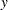COORDINATES Statement
COORDINATES | COORD coordinate-variables ;

The following two options specify the names of the variables in the DATA= data set that contains the values of theandcoordinates of the data.

Only one COORDINATES statement is allowed, and it is applied to all PREDICT statements. In other words, it is assumed that all the VAR= variables in all PREDICT statements have the sameandcoordinates.

This is not a limitation. Since each VAR= variable is processed separately, observations for which the current VAR= variable is missing are excluded. With the next VAR= variable, the entire data are read again, this time excluding missing values in this next variable. Hence, a single run of PROC KRIGE2D can be used for variables measured at different locations without overlap.

XCOORD= (variable-name)
XC= (variable-name)

specifies the name of the variable that contains thecoordinate of the data locations in the DATA= data set.

YCOORD= (variable-name)
YC= (variable-name)

specifies the name of the variable that contains thecoordinate of the data locations in the DATA= data set.